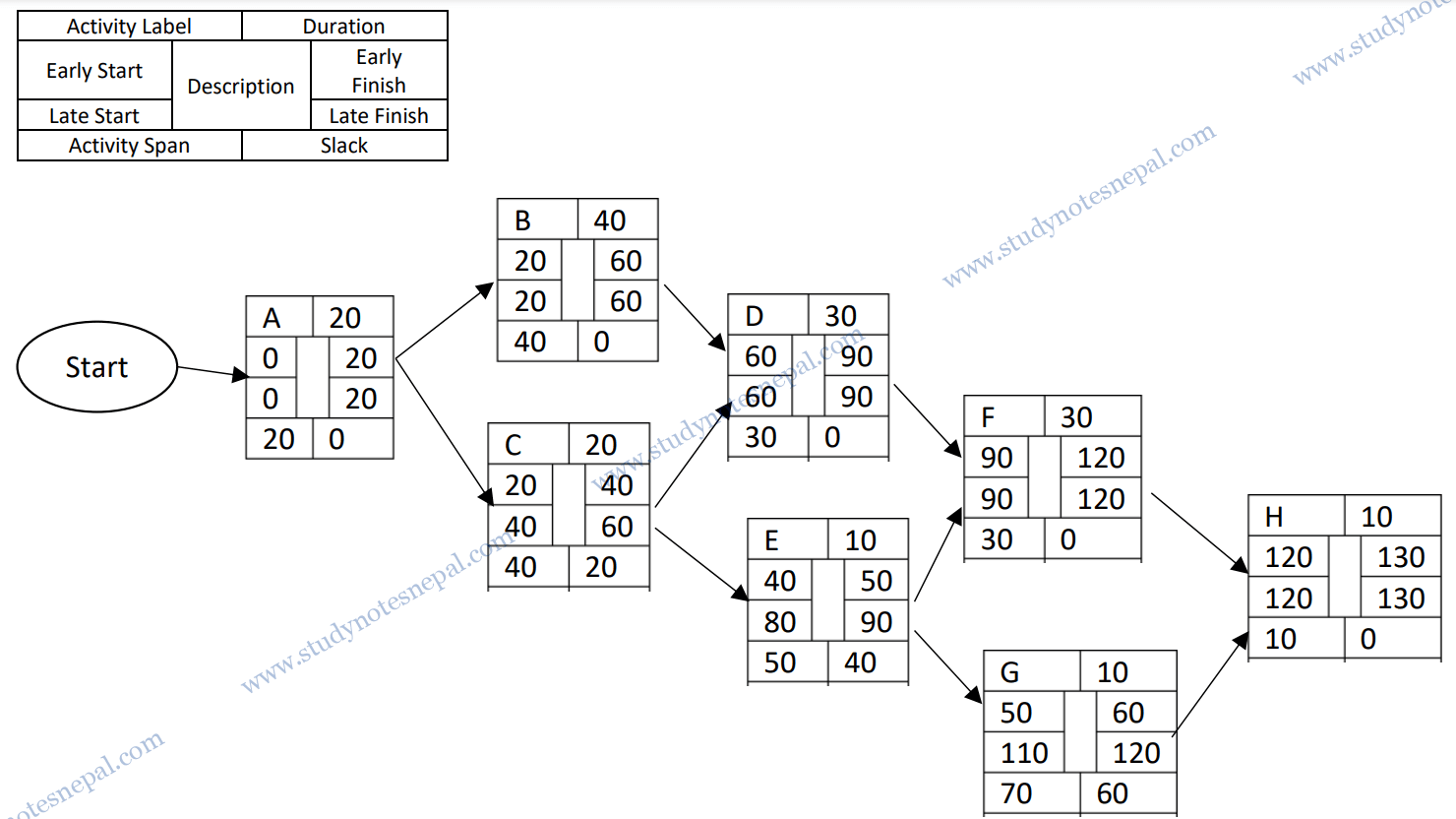What is the Cost Performance Index (CPI)? The Cost Performance Index (CPI) is a method for calculating the cost efficiency and financial effectiveness of a specific project through the following formula: CPI = earned value (EV) / actual cost (AC). A CPI ratio with a value higher than 1 indicates that a project is performing well budget-wise. A CPI value of 1 indicates that a project is performing on budget. A CPI value that is less than 1 indicates that a project is over budget. What is the  Schedule Performance Index (SPI)? The schedule performance index (SPI) is a measure of how close the project is to being completed compared to the schedule. Schedule Performance Index (SPI) = Earned Value (EV) / (PV) If the ratio has a value higher than 1 this indicates the project is progressing well against the schedule. If the SPI is 1, then the project is progressing exactly as planned. If the SPI is less than 1 then the project is running behind schedule.

 Year 2021(Makeup)

Question No. 6: Suppose we are managing a software development project. The project is expected to be completed in 8 months at a cost of \$10,000 per month. After 2 months, you realize that the project is 30 percent completed at a cost of \$40,000. You need to determine whether the project is on-time and on-budget after 2 months.

Solution:

Step 1: Calculate the Planned Value (PV) and Earned Value (EV)

From the scenario,

Budget at Completion (BAC) = \$10,000 * 8 = \$80,000

Actual Cost (AC) = \$40,000

Planned Completion = 2/8 = 25%

Actual Completion = 30%

Therefore,

Planned Value = Planned Completion (%) * BAC = 25% * \$ 80,000 = \$ 20,000

Earned Value = Actual Completion (%) * BAC = 30% * \$ 80,000 = \$ 24,000

Step 2: Compute the Cost Performance Index (CPI) and Schedule Performance Index (SPI)

Cost Performance Index (CPI) = EV / AC = \$24,000 / \$40,000 = 0.6

Schedule Performance Index (SPI) = EV / PV = \$24,000 / \$20,000 = 1.2

Interpretation: Since Cost Performance Index (CPI) is less than one, this means the project is over budget. For every dollar spent we are getting 60 cents’ worth of performance. Since Schedule Performance Index (SPI) is more than one, the project is ahead of schedule. However, this has come at a cost of going over budget. If work is continued at this rate, the project will be delivered ahead of schedule and over budget. Therefore, corrective action should be taken.

Apart from computing the Cost Performance Index (CPI) and Schedule Performance Index (SPI), we can calculate the earned value cost and schedule variance.

Question No. 1: Assume that the size of an organic type software product has been estimated to be 32,000 lines of source code. Assume that the average salary of software engineers be Rs. 15,000/- per month. Determine the effort required to develop the software product and the nominal development time and the development cost.

1. Effort = a (KLOC)b. person month
2. Development Time = c (Effort)d months
3. Development cost= Development Time * Average Salary

Solution:

Given that,

Estimated Lines of Code = 32,000 = 32 KLOC

We have,

Effort = a (KLOC)b. person month

= 2.4(32) 1.05 person month

= 91.3311 person month

= 91 person month

Development Time = c (Effort) d Months

= 2.5 (91) 0.38 Months

=  13.879 Months

= 14 Months

Cost required to develop the product = Development Time * Average Salary

= 14 х 15,000 =

= Rs. 210,000/-

 Year 2021

Question No. 3: Draw a precedence network diagram by performing forward and backward passes. Also determine the critical path and completion date of the project. (Use the information given in the table).

 Task Precedents Duration (Weeks) A – 20 B A 40 C A 20 D C, B 30 E C 10 F D, E 30 G E 10 H F, G 10

Solution:Question No. 5: Suppose you are managing a software development project. The project is expected to be completed in 10 months at a cost of Rs 50,000 per month. After 4 months, you realize that the project is 45 percent completed at a cost of Rs 200,000. You need to determine whether the project is on-time and on budget after 4 months?

Solution:

Step 1: Calculate the Planned Value (PV) and Earned Value (EV)

From the scenario,

Budget at Completion (BAC) = Rs. 50,000 * 10 = Rs. 500,000

Actual Cost (AC) = Rs. 200,000

Planned Completion = 4/10 = 40%

Actual Completion = 45%

Therefore,

Planned Value = Planned Completion (%) * BAC = 40 % * Rs. 500,000 = Rs. 200,000

Earned Value = Actual Completion (%) * BAC = 45% * Rs. 500,000 = Rs. 225,000

Step 2: Compute the Cost Performance Index (CPI) and Schedule Performance Index (SPI)

Cost Performance Index (CPI) = EV / AC = Rs. 225,000 / Rs. 200,000 = 1.125

Schedule Performance Index (SPI) = EV / PV = Rs. 225,000 / Rs. 200,000 = 1.125

Interpretation: Since Cost Performance Index (CPI) is more than one, this means the project is under budget. Since Schedule Performance Index (SPI) is more than one, the project is ahead of schedule.

 Year 2016

Question No. 17: Draw the activity-On-Arrow diagram and calculate project duration and show the critical path with the forward and backward rule.

 Activity Precedence Duration (Months) P None 10 Q None 4 R P 3 S Q 5 T R 1 U Q 5 V None 4 W T, V 6 X R 7 Y X, U 3

Solution: